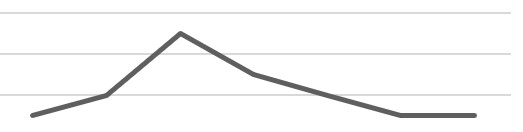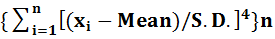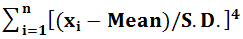# Kurtosis

## Introduction

• Raw data is of no use until it gives some information. To obtain information from data, it should be well sorted to do any kind of analysis. There are many ways to sort and represent data like pictorial representation using charts, frequency distribution charts, etc.
• Symmetric Distribution is one in which frequencies of the elements of the dataset are evenly/equally distributed around the Median or a dataset is said to be symmetric if it looks the same to the left and right of the center point. The following image is an example of how Symmetric Distribution looks, the center point is the Median of the dataset.• A Skewed Distribution is just the opposite of Symmetric Distribution. In this distribution, the frequencies are not well spread around the median. The following image is an example of how a Skewed Distribution looks.• The topmost point in both the images is called as Peak of the Distribution.

## Kurtosis

• Kurtosis is a way to measure the peak of the frequency distribution of a dataset, it gives the measurement in number form, for e.g. Kurtosis of a Normal/Symmetric Distribution is 3.
• The value of Kurtosis can be expressed either as Absolute or Relative value, wherein the Absolute value is always Positive.

### Measurement of Kurtosis

• Kurtosis can be calculated using the following Formula:
• Kurtosis =• Where, xi is the ith element of the dataset, Mean is the Mean of dataset S.D. is the standard deviation of the dataset that has total n elements.
• The value obtained by the above formula is Absolute Kurtosis.
• Relative Kurtosis = Absolute Kurtosis -3
• Most of the time, Kurtosis is expressed in Relative Terms, hence Kurtosis implies relative Kurtosis.

## Types of Kurtosis

• Kurtosis is divided into three types depending upon the absolute value of Kurtosis, the three types are:
• Leptokurtic: If the value of Kurtosis >3, it is known as Leptokurtic and the Distribution Curve is more peaked than the normal.
• Mesokurtic: If the value of Kurtosis = 3, it is known as Mesokurtic and the Distribution Curve is having a normal peak.
• Platykurtic: If the value of Kurtosis <3, it is known as Platykurtic and the Distribution Curve is less peaked than the normal.

#### Example 1:

• Consider a dataset: 2,3,5,6. Calculate the Kurtosis for the dataset if the Standard Deviation is given to be 1.58.
• Solution: Dataset: 2,3,5,6; Number of elements (n) = 4; SD = 1.48
• Mean = (2+3+5+6)/4 = 16/4
• Kurtosis =• Calculating:• = {[ (2 - 4) + (3 - 4) + (5 - 4) + (6 - 4)]/ 1.48}4
• = ( -2)4 + (-1)4  + 14 + 24 / (1.58)4
• = 34/6.23 = 5.45
• Dividing above result by n = 4
• Hence, Kurtosis = 5.45/4 = 1.36